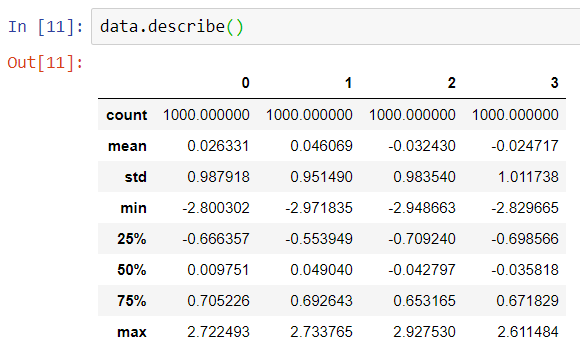Prerequisite: What are Outliers & What is Outlier Detection?

There are several methods of Outlier Detection in data. The most simple method of detecting and filtering outliers from numerical data is to check the difference between the mean and max/min values of the attribute. If the difference is very large, necessarily there are outliers in our dataset.

Let us begin by generating a small dataset. We will generate a pandas data frame of 4 attributes and 1000 rows.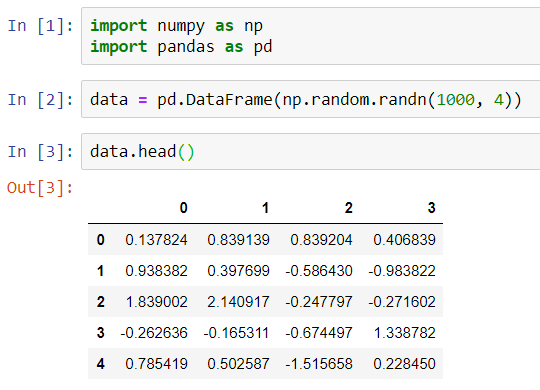Let us check the difference between the mean and max values in each column.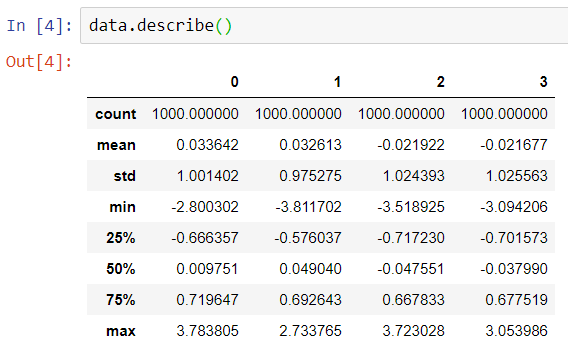We have both positive and negative values in the dataset. We can see from the above description of data that the difference between the (mean and max) and (mean and min) values is huge. Hence, possibly there are outliers in our dataset.

#### Filtering Outliers from Data

There are a lot of ways by which we can filter out outliers from the data. The most simple method is to replace the outliers with the mean values of the respective attributes.

Let us suppose that all the values above 3 and below -3 are outliers. Now, we want to replace all such values with the mean of the respective attributes.

Note that we have talked about absolute values in the analysis below.

Let us first print all the rows where the absolute value of at least any one attribute is greater than 3.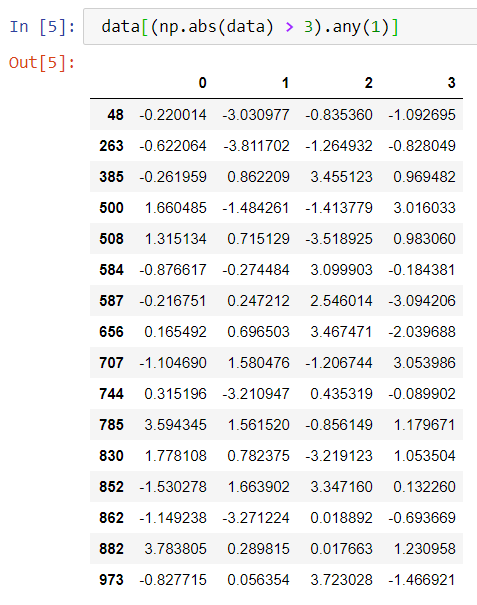Now, for all the above rows, we want to replace the absolute values > 3 with the mean of the respective attribute.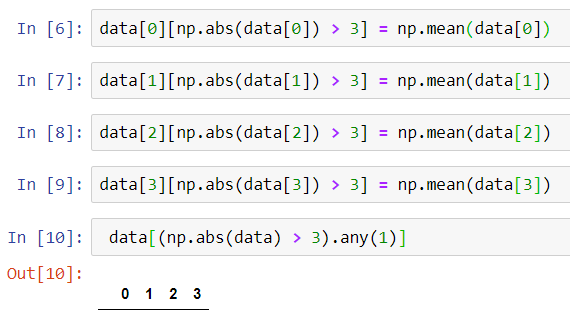We can see from above that now our dataset does not have any column value, where absolute value is greater than 3.

At last, let us once again check the description of the data.